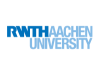list 7 séquences
assignment Niveau : Intermédiaire
chat_bubble_outline Langue : Anglais
Avis de la communauté
-
starstarstarstarstar
Voir l'avis

## Les infos clés

credit_card Formation gratuite
verified_user Certification payante
timer 56 heures de cours

## En résumé

This course will introduce you to a broad range of methodologies used in the field of machine dynamics. You will learn how to model a vehicle using the fundamentals of mechanics. You will get a deep understanding of the equations of motion and how to solve them using powerful MathWorks tools. Eventually, you will gain the ability to analyze and interpret the computational results in order to optimize your design.

To get the most out of your time the course is subdivided into five weeks, each consists of lectures, tutorials and exercises. During lectures, you will get all the theoretical background of machine dynamics. Tutorials will teach you the basics of MathWorks products and exercises will merge your theoretical knowledge with the practical use of the software into an exciting application.

You will learn how to model a vehicle by a one and a two degree of freedom system. These systems could be base excited, force excited or not excited, they could be damped or undamped and their mathematical representation could be solved analytically, by state space representation or by solving the differential equation itself.

Therefore, if you ever wondered how to design a vehicle suspension using MathWorks tools, we highly recommend you attend to this course.

• Basic and advanced MATLAB skills
• Define a sensible modelling depth
• Derive equations of motion for different models
• Solve the equations of motion using different approaches
• Presentation of results using animations

more_horiz Lire plus
more_horiz Lire moins
report_problem

## Les prérequis

• Basics of solid mechanics
• Linear differential equations of second order
• Basics of linear algebra

dns

## Le programme

Week1: Basics of oscillation
Definition of important parameters of an oscillation. Introduction to the methods of
modelling and discussion about modelling depth. Derivation of an equation of motion of a
translational one degree of freedom system.

Week2: Discrete systems with one degree of freedom and its eigen behavior
Analytical solution of an equation of motion and animation of the results. Analysis of
three typical cases of eigen behavior. Introduction to state space representation and
numerical solution of an equation of motion. Getting started with Simulink.

Week3: Discrete systems with one degree of freedom with forced excitation
Description of different types of excitation. Derivation and solution of an equation of
motion with frequency dependent force excitation and frequency independent force
excitation, respectively. Extension of the model to base excitation and explanation and
development of effective vibration insulation.

Week4: Discrete systems with multiple degrees of freedom and its eigen behavior
Derivation of a system of equations of motion which describes vertical dynamics and
pitch motion. Analytical solution of this system and discussion of the homogeneous
solution. Analyzes of three typical cases of motion.

Week5: Discrete systems with multiple degrees of freedom and its eigen behavior
and with forced excitation
Transfer of equations into state space representation and into a Simulink model.
Application of frequency independent and frequency dependent force excitation to the
system of equations of motion. Adaption of the model to base excitation. Development of
a vibration absorber.

Week6: Discrete systems with multiple degrees of freedom with forced excitation
Adaption of the model to base

record_voice_over

## Les intervenants

Burkhard Corves
Univ.-Prof. Dr.-Ing. Dr. h. c.
RWTH Aachen University

Martin Lankers
M.Sc.
RWTH Aachen University

Jan-Lukas Archut
M.Sc.
RWTH Aachen University

store

## Le concepteurThe RWTH Aachen University
assistant

## La plateformeEdX est une plateforme d'apprentissage en ligne (dite FLOT ou MOOC). Elle héberge et met gratuitement à disposition des cours en ligne de niveau universitaire à travers le monde entier. Elle mène également des recherches sur l'apprentissage en ligne et la façon dont les utilisateurs utilisent celle-ci. Elle est à but non lucratif et la plateforme utilise un logiciel open source.

EdX a été fondée par le Massachusetts Institute of Technology et par l'université Harvard en mai 2012. En 2014, environ 50 écoles, associations et organisations internationales offrent ou projettent d'offrir des cours sur EdX. En juillet 2014, elle avait plus de 2,5 millions d'utilisateurs suivant plus de 200 cours en ligne.

Les deux universités américaines qui financent la plateforme ont investi 60 millions USD dans son développement. La plateforme France Université Numérique utilise la technologie openedX, supportée par Google.

Vous êtes le concepteur de ce MOOC ?
Quelle note donnez-vous à cette ressource ?
Contenu
5/5
Plateforme
5/5
Animation
5/5